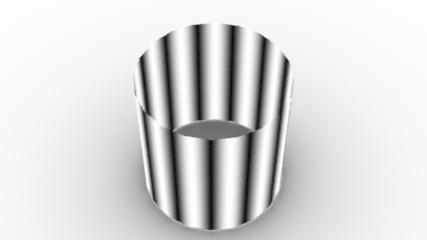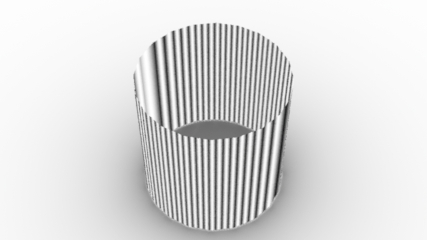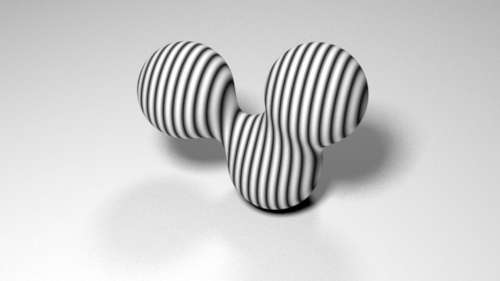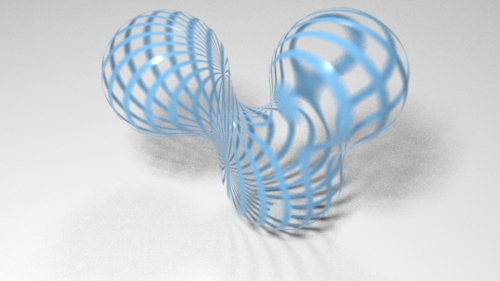### OSL3D Textures

#### Introduction

The features of shading points, such as their position, color and presence, are often determined by their location in the 'st' texture space. For example, listing 1 shows a surface shader that uses the sin() function, based on 's', to generate a cyclic pattern - figure 1.

Listing 1 (simple_sine.osl)

 ```shader simple_sine(float freq = 10, float s = 0 [[int lockgeom = 0]], float t = 0 [[int lockgeom = 0]], output color resultRGB = 0) { float sinval = sin(s * 2 * M_PI * freq); // Remap from -1 to +1 to 0 to 1 resultRGB = (sinval + 1) * 0.5; }```Figure 1
Polygonal cylinder with well defined 'st' coordinates.

Of course, if the shader is attached to geometry that has not been assigned texture coordinates the result of using 's' becomes problematic - figure 2.Figure 2
Polygonal cylinder with undefined texture coordinates.

Even more unpredictable are geometries, such as a Blobby, that cannot be given texture coordinates - figure 3.Figure 3
Blobby primitives cannot be assigned texture coordinates

The next section demonstrates that shading according to 'xyz' position, rather than texture coordinates, generates a 3D solid texture.

#### A 3D Sinusoidal Texture

Rather than calculating a sine value based on a texture coordinate, such as 's', the polar coordinate of a shading point (generally a micro-polygon) can be used.Figure 4

For example, the angular position (theta) of `sp` shown in figure 4 can be specified by its 2D Polar coordinate. For the purpose of creating a pattern of vertical stripes the height of `sp` can be ignored. The shader given in listing 2 uses the sine pattern to define an output color as well as a output float that can be used to control "presence".

Listing 2 (sine3d.osl)

 ```shader sine3d(float freq = 4, float amp = 0.5, float offset = 0, float blur = 0.05, string spacename = "object", output float resultF = 0, output color resultRGB = 0) { // Convert the shading point to the object coordinate system. point p = transform(spacename, P); // Get the coordinates of the shading point. float x = p; float z = p; // The arc tangent of the shading point will be in radians // (-3.142 to 3.142) ie -180 to 180 degress. float theta = atan2(x,z); // Generate a sine wave - remapped to 0 to 1. float wave = (sin(theta * freq) + 1) * 0.5; // Adjust the height and position of the sine wave. resultF = wave * amp + offset; // Get a filtered value of the wave height. wave = smoothstep(resultF - blur, resultF + blur, p); // Control the mixing of two colors by the wave. resultRGB = mix(color(1,1,1), color(0,0,0), wave); } ```

Figure 5 shows the sine3d shader controlling the color of a blobby.Figure 5 ``` Pattern "PxrOSL" "sine3d4" "string shader" "sine3d" "float freq" 34 "float amp" 2 "float offset" -1 "float blur" 0.05 "string spacename" ["object"] Bxdf "PxrDisney" "PxrDisney1" "reference color baseColor" ["sine3d4:resultRGB"] "float specular" [0.5]```

 Figure 6 shows the sine3d shader controlling the presence of a blobby.Figure 6 ``` Pattern "PxrOSL" "sine3d4" "string shader" "sine3d" "float freq" 34 "float amp" 2 "float offset" -1 "float blur" 0.05 "string spacename" ["object"] Bxdf "PxrDisney" "PxrDisney1" "float specular" [0.5] "reference float presence" ["sine3d4:resultF"]```